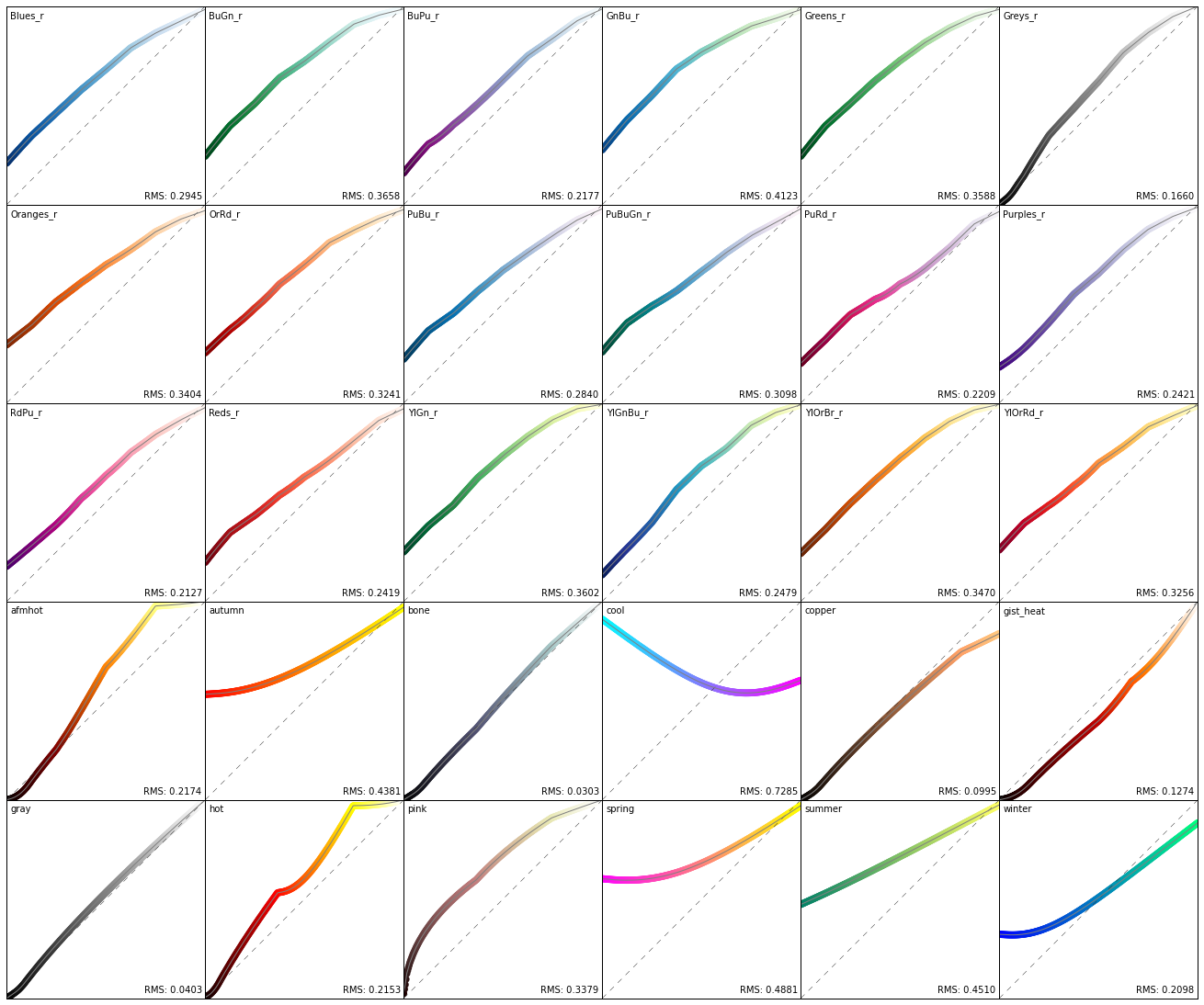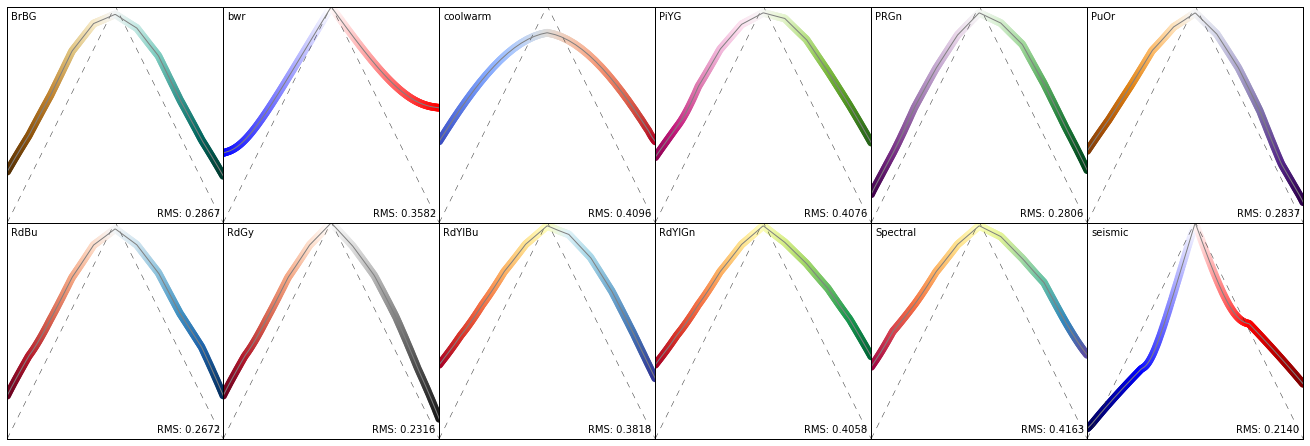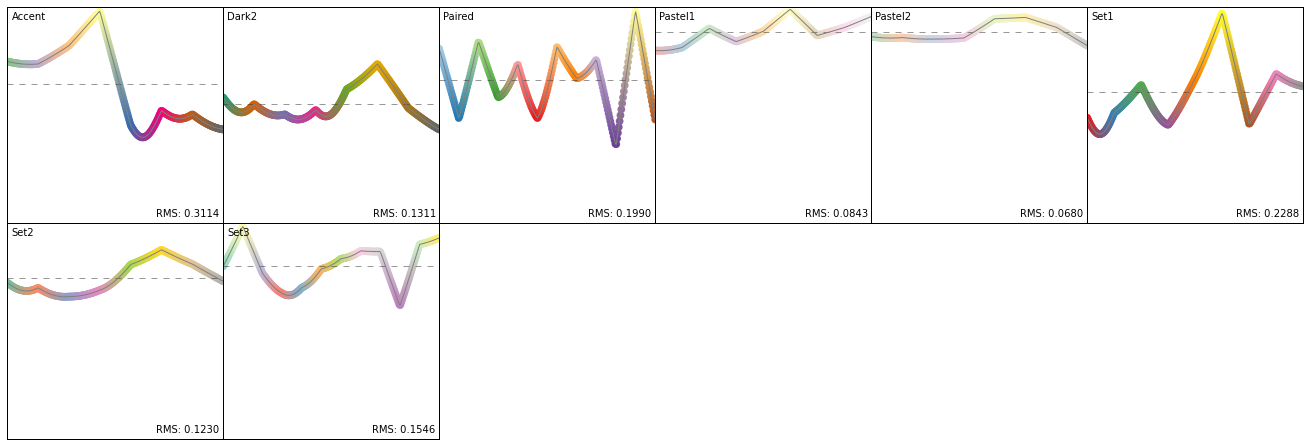# Perceptually Uniform Colormaps in Matplotlib¶

This notebook aims to explore and illustrate the performance of matplotlib's colormaps for qualitative plots. The most vital property of any graph is that humans are able to interpret and judge it. For plotting a collection of values this means that the perceived difference between any two values should equal the actual difference between these values. Turns out the jet colormap (matplotlib's default) is pretty terrible at this, as are many other colormaps.

The main reason for this is that computers usually work in the RGB color space, distances in which is are not perceptually uniform for humans. The LAB colorspace on the other hand is designed to approximate human vison. One axis is the lightness which is supposed to be linearly related to the perceived brightness. Therefore if a range of values is linearly mapped to the lightness of some colors we should be good. In this notebook we will use this as a criteria for the goodness of a colormap. Another advantage of linear lightness colormaps is that their grayscale representations are still usable which even nowadays is important for printing.

Please be aware that I have only a limited understanding of colorspaces and related concepts and don't really know what I am talking about, but there are a great number of resources out there, e.g.:

In :
%pylab inline
from __future__ import print_function

import matplotlib
import matplotlib.pyplot as plt
import numpy as np

# Scikit-images has a bug when converting colors from LAB to RGB:
# https://github.com/scikit-image/scikit-image/issues/1201
# Thus it cannot be used here.
# import skimage.color
from colormath.color_objects import LabColor, sRGBColor
from colormath.color_conversions import convert_color

Populating the interactive namespace from numpy and matplotlib


All colormaps shipping with matplotlib, from http://matplotlib.org/examples/color/colormaps_reference.html

Sequential ones are merged and partially reversed.

In :
cmaps = {'sequential':     ['Blues_r', 'BuGn_r', 'BuPu_r',
'GnBu_r', 'Greens_r', 'Greys_r', 'Oranges_r', 'OrRd_r',
'PuBu_r', 'PuBuGn_r', 'PuRd_r', 'Purples_r', 'RdPu_r',
'Reds_r', 'YlGn_r', 'YlGnBu_r', 'YlOrBr_r', 'YlOrRd_r',
'afmhot', 'autumn', 'bone', 'cool', 'copper',
'gist_heat', 'gray', 'hot', 'pink',
'spring', 'summer', 'winter'],
'diverging':      ['BrBG', 'bwr', 'coolwarm', 'PiYG', 'PRGn', 'PuOr',
'RdBu', 'RdGy', 'RdYlBu', 'RdYlGn', 'Spectral',
'seismic'],
'qualitative':    ['Accent', 'Dark2', 'Paired', 'Pastel1',
'Pastel2', 'Set1', 'Set2', 'Set3'],
'misc':           ['gist_earth', 'terrain', 'ocean', 'gist_stern',
'brg', 'CMRmap', 'cubehelix',
'gnuplot', 'gnuplot2', 'gist_ncar',
'nipy_spectral', 'jet', 'rainbow',
'gist_rainbow', 'hsv', 'flag', 'prism']}

In :
def optimal_lightness(npts, cmap_type, l_range=(0.0, 1.0)):
"""
Helper function defining the optimality condition for a colormap.
Depending on the colormap this might mean different things. The
l_range argument can be used to restrict the lightness range so it
does have to equal the full range.
"""
if cmap_type == "sequential_rising":
opt = np.linspace(l_range, l_range, npts)
elif cmap_type == "sequential_falling":
opt = np.linspace(l_range, l_range, npts)
elif cmap_type == "diverging_center_top":
s = npts // 2
opt = np.empty(npts)
opt[:s] = np.linspace(l_range, l_range, s)
opt[s:] = np.linspace(l_range, l_range, npts - s)
elif cmap_type == "diverging_center_bottom":
s = npts // 2
opt = np.empty(npts)
opt[:s] = np.linspace(l_range, l_range, s)
opt[s:] = np.linspace(l_range, l_range, npts - s)
elif cmap_type == "flat":
opt = np.ones(npts) * l_range
return opt

In :
def plot_cmap_lightness(name, ax, cmap_type=None, l_range=(0.0, 1.0)):
"""
Plot the perceived lightness vs position in the colormap.
"""
cmap = plt.get_cmap(name)

x = np.linspace(0, 1, 256)
values = cmap(x)[:, :3]

lab_colors = []
for rgb in values:
lab_colors.append(convert_color(sRGBColor(*rgb), target_cs=LabColor))
lightness = [_i.lab_l for _i in lab_colors]

if cmap_type == "flat":
mean = np.mean(lightness) / 100.0
optimality = optimal_lightness(len(x), cmap_type=cmap_type, l_range=(mean, mean))
else:
optimality = optimal_lightness(len(x), cmap_type=cmap_type, l_range=l_range)

# Plot reference.
ax.plot(x * 100.0, optimality * 100, color="0.2", lw=0.5, ls="--")

ax.scatter(x * 100.0, lightness,
c=x, cmap=cmap, edgecolor="None",
s=60)

# Calculate "RMS Misfit" as defined by Gellar and Takeuchi 1995.
rms = np.sqrt(
np.sum((np.array(lightness) - (optimality * 100)) ** 2) / np.sum((x * 100) ** 2))

ax.plot(x * 100.0, lightness, c="0.5", lw=1)
ax.set_xlim(0, 100)
ax.set_ylim(0, 100)
ax.set_aspect("equal")
ax.text(0.02, 0.98, cmap.name, horizontalalignment='left',
verticalalignment='top', transform=ax.transAxes)
ax.text(0.98, 0.02, "RMS: %.4f" % rms, horizontalalignment='right',
verticalalignment='bottom', transform=ax.transAxes)
ax.get_xaxis().set_visible(False)
ax.get_yaxis().set_visible(False)


### Sequential Colormaps¶

Plots the lightness in the LAB color space for each colormap against the position in the colormap. The ideal curve is the dashed grey line. RMS is the root mean square misfit between the actual curve and the ideal one.

In :
plt.figure(figsize=(18, 15))
gs = matplotlib.gridspec.GridSpec(5, 6, left=0.0, right=1.0, bottom=0.0,
top=1.0, wspace=0.0, hspace=0.0)
for g, cmap in zip(gs, cmaps["sequential"]):
plot_cmap_lightness(cmap, plt.subplot(g), cmap_type="sequential_rising")
plt.show()### Diverging Colormaps¶

In :
plt.figure(figsize=(18, 6))
gs = matplotlib.gridspec.GridSpec(2, 6, left=0.0, right=1.0, bottom=0.0,
top=1.0, wspace=0.0, hspace=0.0)
for g, cmap in zip(gs, cmaps["diverging"]):
plot_cmap_lightness(cmap, plt.subplot(g), cmap_type="diverging_center_top")
plt.show()### Quantitative Colormaps¶

In :
plt.figure(figsize=(18, 6))
gs = matplotlib.gridspec.GridSpec(2, 6, left=0.0, right=1.0, bottom=0.0,
top=1.0, wspace=0.0, hspace=0.0)
qm = len(cmaps["qualitative"])
for g, cmap in zip([gs[_i] for _i in range(qm)], cmaps["qualitative"]):
plot_cmap_lightness(cmap, plt.subplot(g), cmap_type="flat")
plt.show()### Misc Colormaps¶

This, amongst other things, illustrates how terrible the default jet colormap actually is for quantitative plots.

In :
plt.figure(figsize=(18, 9))
gs = matplotlib.gridspec.GridSpec(3, 6, left=0.0, right=1.0, bottom=0.0,
top=1.0, wspace=0.0, hspace=0.0)
mm = len(cmaps["misc"])
for g, cmap in zip([gs[_i] for _i in range(mm)], cmaps["misc"]):
plot_cmap_lightness(cmap, plt.subplot(g), cmap_type="sequential_rising")
plt.show()